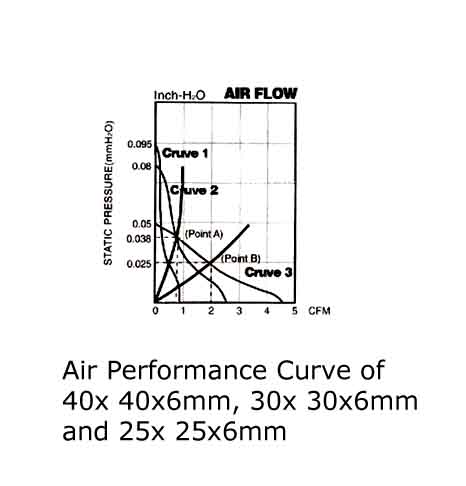# How to select the right fan or blower for your application

While designing electrical and electronic appliances the engineers need to determine the air flow required for dissipating heat. To prevent overheating, the air flow value required depends on power consumption in the system to make sure it takes away enough heat. The facts show that the service life of a system will decline due to an insufficient cooling system, sales and price may decrease because the lifetime does not meet the user's expectations.

To select a right heat dissipation device, the following objectives have to be considered:

l  efficient air flow

l  Minimum size and fit

l  Minimum noise

l  Minimum power consumption

l  Reliable and long service life

l  Reasonable cost

Here are three essential steps to select the right fan or blower for your application to achieve the above objectives.

Step 1. Total cooling Requirements

The first step is to recognize three key factors to obtain the total cooling requirements:

l  The heat that has to be transferred:  difference of temperatures (DT)

l  Electrical power (W) to offset the transferred heat

l  Airflow required to remove heat (CFM)

The total cooling requirements are very important for a system to operate effectively. An efficient operating system is to provides the desired operating conditions for all components in the system to maximize the performance and service life.

The following methods could be used to select fan motors:

l  Determine the amount of heat generated inside the equipment

l  Determine the permissible range of temperature rise inside the equipment.

l  Calculate the air volume required from the equation.

l  Estimate system impedance of the equipment.

l  Select the fan according to the performance curve shown in the catalog or datasheet.

The airflow volume required to cool the equipment can be obtained, if the internal heat dissipation and the permissible total rising temperature are known.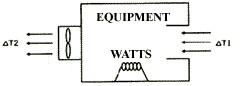The following is the basic heat transfer equation:

H = Cp × W × △ T

H = Amount of heat transferred

CP = specific heat of air

Δ t = temperature rise in the cabinet

W = Mass flow

We have W = CFM × D, where D = air density.

After substitution, we get

Q(CFM)=Q / ( Cp * D * Δ T )

By incorporating the conversion factors and the specific heat and density of the sea-level air, the following heat dissipation equation can be obtained: CFM = 3160 × KW / △℉

Then the following equation is obtained:

Q(CFM）=3.16 * P / Δ Tf=1.76 * P / Δ Tc

Q(M3/Min)=0.09 * P / Δ Tf=0.056 * P / Δ Tc

Q: Air flow required for cooling

P: Heat dissipation inside the cabinet (i.e., electrical power consumed by the equipment)

TF: allowable internal temperature rises in Fahrenheit

TC: allowable internal temperature rises in Centigrade

DT = temperature difference between DT1 and DT2

Example 1: The internal power consumption of the equipment is 500 watts, and the temperature difference is 20 ° F. the following are the calculation results:

Q=3.16*500W/20=79CFM or Q=0.09*500W/20=2.25M3/Min

Example 2: the internal power consumption of the equipment is 500W, and the temperature difference is 10 ℃

Q=1.76*500W/10=88CFM or Q=0.056*500W/10=2.8M3/Min

Step 2: total system impedance / system characteristic curves

When the air flows, it will encounter obstructions of the parts inside the cabinet in its flow path, and the impedances will limit the air flows freely. The change in pressure is static pressure in inches of water.

In order to confirm the cooling wattage of each slot, the system designer or manufacturer must not only have the characteristic curve of a fan to determine the maximum air flow but must also know the air resistance curve of the system. There is a loss of air pressure due to the resistance of components inside the cabinet. This loss varies with air flow and is known as system resistance.

The system characteristic curve is defined as follows:

DP= KQn

K = system characteristic coefficient

Q = air flow (cubic feet)

N = disturbing factor, 1 < n < 2

For stratospheric flow, n = 1

In turbulent flow, n = 2

Step 3: system operating point

The intersection of the system impedance curve and air flow characteristic curve is called the System Operating Point, which is the best operating point of the fan for your application.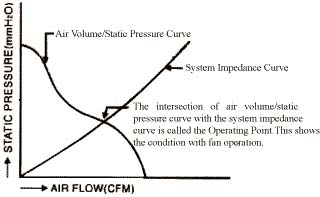At the operating point, the change slope of the fan characteristic curve is minimized while the change rate of the system characteristic curve is at the lowest.

Note that the static efficiency (air flow times static pressure divided by power consumption) of the fan is optimized also.

Designing Considerations:

1. Keep the air flow path as unobstructed as possible, and keep the air inlet and outlet unblocked for air flow.

2. Guide the air flows throusgh the system vertically to ensure air flows smoothly and improve cooling efficiency.

3. If an air filter is necessary, the additional resistance to air flow should be considered

Examples of selecting the best fan for your application:

### Example 1.

Figure #1 is an air characteristic curve of DC cooling fan 60 × 60 × 25 mm. The fan might be applied at Point A or Point C, delivering 6 CFM or 20 CFM respectively, if the system resistance were imposed a pressure drop of 0.16(at Point A) or 0.04(at Point C) Inch-H2O on the air stream. If the system operates at point B due to improvement, the fan might be delivering 12cfm at a pressure of only 0.09 Inch- H2O.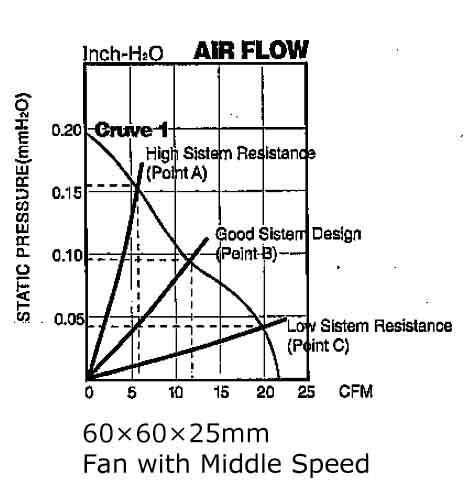### Example 2.

As shown in Figure #2, Characteristic Curve 2 is a fan of the same size and shape, but lower speed than Characteristic Curve 1. If the system only requires 15CFM at 0.05 Inch-H2O, the intersection of static pressure and air flow curve should be through point B. Therefore, the fan provides 18CFM at zero static pressure, which is enough for cooling. Thus, the final arrangement is to choose a fan of lower speed.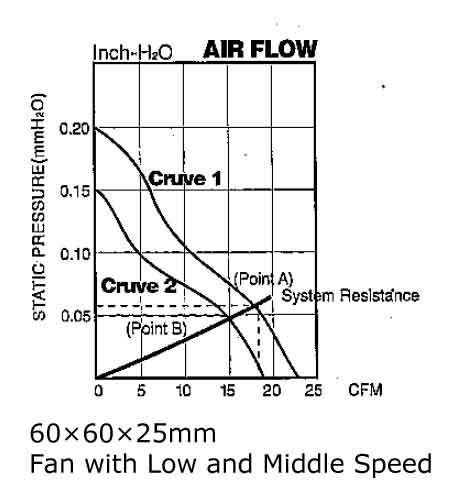### Example 3.

Figure #3 shows the air performance curves of 40 × 40 × 6mm (Curve 3), 30 × 30 × 6mm (Curve 2), and 25 × 25 × 6 mm (Curve 1) medium speed DC fan.

Case 1:

If the system impedance is 0.025 Inch-H2O and 2 CFM air flow is required for cooling, 40 × 6mm DC fan is recommended（ Please refer to point B)

Case 2:

If more components are added to the system and/or there is a more compact physical re-configuration, a higher system impedance will be generated. Now assume that the system impedance rises to 0.038 Inch-H2O and requires 0.85 CFM for cooling. There are two fans available for selection: 40 x 6mm and 30 x 6mm. (Refer to Operating Point A). Another option for cooling a high impedance system is a Micro DC blower.